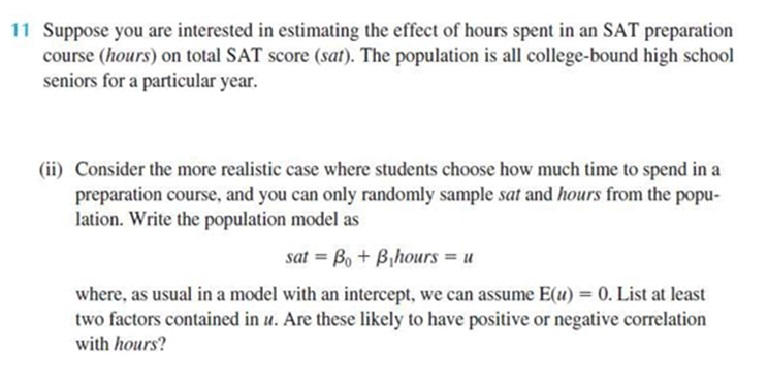Home / Answered Questions / Other / 11-suppose-you-are-interested-in-estimating-the-effect-of-hours-spent-in-an-sat-preparation-course-h-aw177

# (Solved): 11 Suppose You Are Interested In Estimating The Effect Of Hours Spent In An SAT Preparation Course (...11 Suppose you are interested in estimating the effect of hours spent in an SAT preparation course (hours) on total SAT score (sat). The population is all college-bound high school seniors for a particular year. (ii) Consider the more realistic case where students choose how much time to spend in a preparation course, and you can only randomly sample sat and hours from the popu- lation. Write the population model as sat = B. + B hours = u where, as usual in a model with an intercept, we can assume E(u) = 0. List at least two factors contained in u. Are these likely to have positive or negative correlation with hours?

We have an Answer from Expert